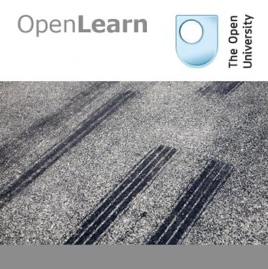1 episode

This unit is the second in the MSXR209 series of five units on mathematical modelling. In this unit you are asked to relate the stages of the mathematical modelling process to a previously formulated mathematical model. This example, that of skid mark produced by vehicle tyres, is typical of accounts of modelling that you may see in books, or produced in the workplace. The aim of this unit is to help you to draw out and to clarify mathematical modelling ideas by considering the example. It assumes that you have studied Modelling pollution in the Great Lakes (MSXR209_1). This study unit is just one of many that can be found on LearningSpace, part of OpenLearn, a collection of open educational resources from The Open University. Published in ePub 2.0.1 format, some feature such as audio, video and linked PDF are not supported by all ePub readers.# Analysing skid marks - for iBooks The Open University

• Courses

This unit is the second in the MSXR209 series of five units on mathematical modelling. In this unit you are asked to relate the stages of the mathematical modelling process to a previously formulated mathematical model. This example, that of skid mark produced by vehicle tyres, is typical of accounts of modelling that you may see in books, or produced in the workplace. The aim of this unit is to help you to draw out and to clarify mathematical modelling ideas by considering the example. It assumes that you have studied Modelling pollution in the Great Lakes (MSXR209_1). This study unit is just one of many that can be found on LearningSpace, part of OpenLearn, a collection of open educational resources from The Open University. Published in ePub 2.0.1 format, some feature such as audio, video and linked PDF are not supported by all ePub readers.

Analysing skid marks

## Analysing skid marks

This unit is the second in the MSXR209 series of five units on mathematical modelling. In this unit you are asked to relate the stages of the mathematical modelling process to a previously formulated mathematical model. This example, that of skid mark produced by vehicle tyres, is typical of accounts of modelling that you may see in books, or produced in the workplace. The aim of this unit is to help you to draw out and to clarify mathematical modelling ideas by considering the example. It assumes that you have studied Modelling pollution in the Great Lakes (MSXR209_1). This study unit is just one of many that can be found on LearningSpace, part of OpenLearn, a collection of open educational resources from The Open University. Published in ePub 2.0.1 format, some feature such as audio, video and linked PDF are not supported by all ePub readers.

5.0 out of 5
1 Rating

1 Rating